## Example Questions

← Previous 1

### Example Question #3 : Geometry

What is the circumference of a circle with an area of 36π?

32

12π

15π

12π

Explanation:

We know that the area of a circle can be expressed: a = πr2

If we know that the area is 36π, we can substitute this into said equation and get: 36π = πr2

Solving for r, we get: 36 = r2; (after taking the square root of both sides:) 6 = r

Now, we know that the circuference of a circle is expressed: c = πd.  Since we know that d = 2r (two radii, placed one after the other, make a diameter), we can rewrite the circumference equation to be: c = 2πr

Since we have r, we can rewrite this to be: c = 2π*6 = 12π

### Example Question #4 : Geometry

Which is greater: the circumference of a circle with an area of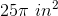, or the perimeter of a square with side lengthinches?

The relationship cannot be determined from the information given.

The two quantities are equal.

The perimeter of the square is greater.

The circumference of the circle is greater.

The circumference of the circle is greater.

Explanation:

Starting with the circle, we need to find the radius in order to get the circumference. Findby plugging our given area into the equation for the area of a circle: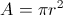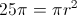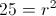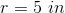Then calculate circumference: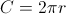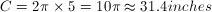(approximatingas 3.14)

To find the perimeter of the square, we can use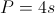, where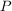is the perimeter andis the side length: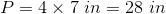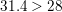, so the circle's circumference is greater.

### Example Question #5 : Geometry

Circle A has an area of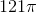.  What is the perimeter of an enclosed semi-circle with half the radius of circle A?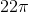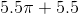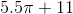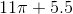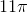Explanation:

Based on our information, we know that the 121π = πr2; 121 = r2; r = 11.

Our other circle with half the radius of A has a diameter equal to the radius of A.  Therefore, the circumference of this circle is 11π.  Half of this is 5.5π.  However, since this is a semi circle, it is enclosed and looks like this: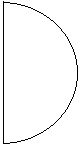Therefore, we have to include the diameter in the perimeter.  Therefore, the total perimeter of the semi-circle is 5.5π + 11.

### Example Question #6 : Geometry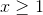Quantity A: The circumference of a circle with radius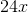Quantity B: The area of a circle with a diameter one fourth the radius of the circle in Quantity A

Which of the following is true?

Quantity B is larger.

The relationship between the two values cannot be determined.

Quantity A is larger.

The two quantities are equal.

The relationship between the two values cannot be determined.

Explanation:

Let's compute each value separately. We know that the radii are positive numbers that are greater than or equal to. This means that we do not need to worry about the fact that the area could represent a square of a decimal value like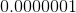.

Quantity A

Since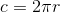, we know: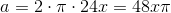Quantity B

If the diameter is one-fourth the radius of A, we know: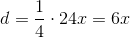Thus, the radius must be half of that, or.

Now, we need to compute the area of this circle. We know:Therefore,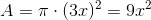Now, notice that if, Quantity A is larger.

However, if we choose a value like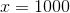, we have:

Quantity A: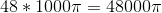Quantity B: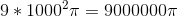Therefore, the relation cannot be determined!

### Example Question #7 : Geometry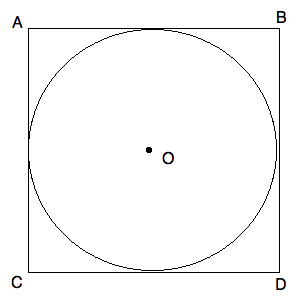Circle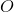has a center in the center of Square.

The area of Squareis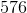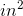.

What is the circumference of Circle?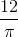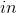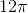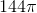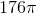Explanation:

Since we know that the area of Squareis, we know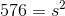, whereis the length of one of its sides. From this, we can solve forby taking the square root of both sides. You will have to do this by estimating upward. Therefore, you know that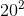is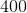. By careful guessing, you can quickly see that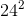is. From this, you know that the diameter of your circle must be half of, or(because it is circumscribed). Therefore, you can draw: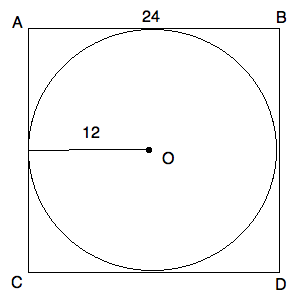The circumference of this circle is defined as:or, for your values: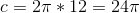(You could also compute this from the diameter, but many students just memorize the formula above.)

### Example Question #8 : Geometry

What is the area of a circle, one-quarter of the circumference of which is 5.5 inches?

225π

π/3

121π

121/π

121/π

Explanation:

Here, you need to “solve backward” from the data you have been given. We know that 0.25C = 5.5; therefore, C = 22. In order to solve for the area, we will need the radius of the circle. This can be obtained by recalling that C = 2πr. Replacing 22 for C, we get 22 = 2πr.

Solve for r: r = 22 / 2π = 11 / π.

Now, we solve for the area: A = πr2.  Replacing 11 / π for r: A = π (11 / π)2 = (121π) / (π2) = 121 / π.

### Example Question #1 : Geometry

In the diagram above, square ABCD is inscribed in the circle.  If the area of the square is 9, what is the area of the circle?

18π

3√(2)π

4.5π

4.5π

Explanation:

If the area of the square is 9, then s2 = 9 and s = 3.  If the sides thus equal 3, we can calculate the diagonals (either CB or AD) by using the 45-45-90 triangle ratio.  For a side of 3, the diagonal will be 3√(2).  Note that since the square is inscribed in the circle, this diagonal is also the diameter of the circle.  If it is such, the radius is one half of that or 1.5√(2).

Based on that value, we can computer the circle’s area:

A = πr2 = π(1.5√(2))2 =  (2.25 * 2)π = 4.5π

### Example Question #10 : Geometry

Quantitative Comparison

Quantity A: Area of a circle with radius r

Quantity B: Perimeter of a circle with radius r

Quantity B is greater.

Quantity A is greater.

The relationship cannot be determined from the information given.

The two quantities are equal.

The relationship cannot be determined from the information given.

Explanation:

Try different values for the radius to see if a pattern emerges.  The formulas needed are Area = πr2 and Perimeter = 2πr.

If r = 1, then the Area = π and the Perimeter = 2π, so the perimeter is larger.

If r = 4, then the area = 16π and the perimeter = 8π, so the area is larger.

Therefore the relationship cannot be determined from the information given.

### Example Question #11 : Geometry

Quantitative Comparison

A circle has a radius of 2.

Quantity A: The area of the circle

Quantity B: The circumference of the circle

Quantity A is greater.

The two quantities are equal.

Quantity B is greater.

The relationship cannot be determined from the information given.

The two quantities are equal.

Explanation:

This is one of the only special cases where the area equals the circumference of the circle. The Area = πr2 = 4π.  The circumference = 2πr = 4π.

Note: For a quantitative comparison such as this one where the columns have numeric values instead of variables, the answer will rarely be "cannot be determined".

### Example Question #11 : Geometry

Quantitative Comparison

Quantity A: Area of a right triangle with sides 7, 24, 25

Quantity B: Area of a circle with radius 5

The relationship cannot be determined from the information given.

The two quantities are equal.

Quantity A is greater.

Quantity B is greater.

Quantity A is greater.

Explanation:

Quantity A: area = base * height / 2 = 7 * 24/2 = 84

Quantity B: area = πr2 = 25π

Now we have to remember what π is.  Using π = 3, the area is approximately 75.  Using π = 3.14, the area increases a little bit, but no matter how exact an approximation for π, this area will never be larger than Quantity A.

← Previous 1

Tired of practice problems?

Try live online GRE prep today.